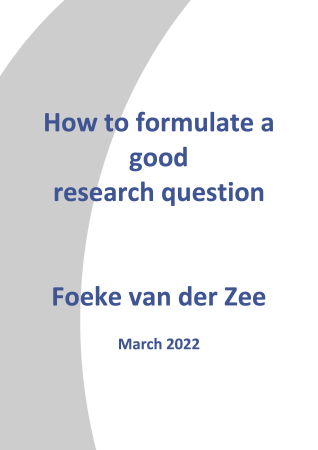Test-test reliabilty# Research-Coaches.com

## Test-test reliability

### Test-test reliability is a statement about the reliability of the information collected by comparing the answers to exactly the same questions.

During an interview, the interviewer can pretend that he did not hear the answer correctly and the question can be repeated. Hopefully the answer is the same. If so, the information is 100% reliable. Small differences can give a slightly different picture of the subject being questioned and this will reduce reliability. This technique can be used in interviews, but is hardly possible in surveys.

It is almost impossible to ask the same question again, or a series of questions over and over again. People will get bored and start giving different answers thinking the given answer is not what the researcher wanted to hear. An alternative is not to use the same questions again but to make use of similar questions. A question might be What is your age? A similar question is What is your day of birth? With this second question the age of the respondent can be calculated and the correlation between these two answers should be 1. If not, one or more people did not give a correct answer.

## The conditions for making a similar test

It is hard to make a similar test with the same content and the same meaning. In theory both tests should have the same mean and the same standard deviation. Maybe this is only possible in a calculation test (1 + 2 = ? is almost similar to 2 + 1 = ?), but questions about the happiness of being married, are a lot more difficult. Nevertheless, give it a try.

Firstly give up the necessary condition to have equal means and equal standard deviations. After all, a correlation coefficient has to be computed and therefore it is unnecessary to have equal means and standard deviations. Secondly, it is not necessary to have exactly the same questions. The score on both tests should be more or less equal and that does not mean the answers to all questions should be equal too. So if two tests are supposed to measure fear of spiders, and the correlation between both test scores is rather high (say over .90) then both tests have a high score for reliability.

Can a correlation between two tests be 1? Yes it can, but that is hardly ever the case. It would mean exactly the same has been measured with different questions, which would be unique. Sometimes this happens with small numbers of respondents or objects but as the number of respondents of objects increases, the correlation might be high, but 1 is never reached.

## A perfect research starts with a good research question.

The biggest cause of a tough ongoing research is a bad research question. Do you have a good one?

Download this E-book for free and discover whether your research question can stand the requirements to become a good research.

50%
To whom can we send it?